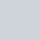# C++ Program to Reverse a Number

Last updated on:

In this tutorial, we will learn about the reverse of a number in C++ i.e. how to write a program to reverse a number using C++.

## Quick Info:💡

↪ Reversing a number means, the digits of a number get reversed i.e. digits of the number are written from right to left.

↪ For example, 342 becomes 243, 45678 becomes 87654 etc.

↪ Here, we will write a C++ code to reverse a number and to display its output in the output console.

↪ Code to reverse a number can be basically performed in two ways:

## Reverse a Number Using While Loop

Here’s a direct method to reverse a number by using while loop.

Simply, the user will be asked to enter a positive integer then, the number is computed and the result is displayed to a user.

### Example:

``````#include<iostream.h>
#include<conio.h>
void main()
{
int num,rev=0,rem,temp;
//you can also take 'long'
cout<<"enter a number:=";
cin>>num;
temp=num;
while(temp>0)
{
rem=temp%10;
rev=rev*10+rem;
temp/=10;
}
cout<<"Reverse of a number is:"<<rev
getch();
}``````
``````//Output:
enter a number:=342
Reverse of a number is:=243``````

### Working:

Flow of a program:

## Reverse a Number Using Function

Functions are used to perform certain actions, and they are important for reusing code: Define the code once and use it many times.

And, this is a another method to find the reverse of the number i.e. by function

Note: Always remember that execution starts from the main body only.

### Example:

``````#include<iostream.h>
#include<conio.h>
int reverse(int num)
{
int rev=0,rem,temp;
temp=num;
while(temp>0)
{
rem=temp%10;
rev=rev*10+rem;
temp/=10;
}
cout<<"Reverse of a number is:"<<rev
return 0;
}
void main()
{
int n;
cout<<"Enter a number:=";
cin>>n;
reverse(n);
getch();
}``````
``````//Output:
Enter a number:=345
Reverse of the number is:=543``````

Well, I hope it really helped you to know C++ better.

Attention reader⚠ Don’t stop learning now.

Want more program on functions, like Armstrong, Palindrome etc.

Just stay with us to get more such exciting codes to explore more in C++.

1.1.